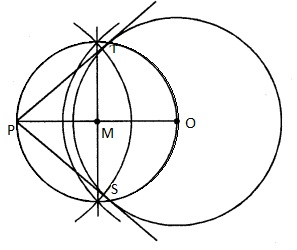# Draw a circle of radius 6 cm. From a point 10 cm away from its centre, construct the pair of tangents to the circle and measure their lengths.

Given:

A circle of radius 6 cm.

To do:

We have to draw a circle of radius 6 cm. From a point 10 cm away from its centre, construct the pair of tangents to the circle and measure their lengths.

Solution:Steps of construction:

(i) Draw a circle with centre $O$ and radius $6\ cm$.

(ii) Take a point $P, 10\ cm$ away from the centre $O$.

(iii) Join $PO$ and bisect it at $M$.

(iv) With centre $M$ and diameter $PO$, draw a circle intersecting the given circle at $T$ and $S$.

(v) Join $PT$ and $PS$.

$PT$ and $PS$ are the required tangents.

Updated on: 10-Oct-2022

49 Views